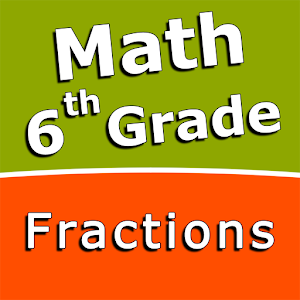Español

Todos los días ofrecemos apliaciones y juegos licenciados GRATIS para Android de otra manera tendrás que comprarlo.\$2.99
EXPIRÓ

# Android Giveaway of the Day - Fractions and mixed numbers - 6th grade math

Add fractions and mixed numbers with like denominators.
\$2.99 EXPIRÓ
Votación de Usuario: 0 (0%) 0 (0%)

Esta oferta de regalo ha expirado. Fractions and mixed numbers - 6th grade math ya está disponible.

Intuitive interface powered by handwriting input and a choice of three fun and engaging mini games in addition to a regular math trainer mode make our app stand out from the crowd of generic math learning apps.

With Fractions and mixed numbers - 6th grade math you can practice and improve the following math skills:
- Write fractions in lowest terms
- Add fractions and mixed numbers with like denominators
- Add fractions and mixed numbers with unlike denominators
- Subtract fractions and mixed numbers with like denominators
- Subtract fractions and mixed numbers with unlike denominators
- Multiply fractions and mixed numbers
- Multiply mixed numbers and whole numbers
- Divide fractions and mixed numbers
- Divide mixed numbers by whole numbers
- Convert fractions and mixed numbers to decimals

Sergey Malugin

Educational

### Versión:

Varies with device

### Tamaño:

Varies with device

Everyone